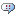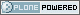# DBAzine.com

##### Personal tools
You are here: Home » Question: "How can I find out if two tables are equal to each other?"
Seeking new owner for this high-traffic DBAzine.com site.
##### Who Are You?
I am a:
Mainframe True Believer
Distributed Fast-tracker

[ Results | Polls ]

# Question: "How can I find out if two tables are equal to each other?"

by Joe Celko

How can I find out if two tables are equal to each other? This is a common programming problem and the specification sounds obvious.

When two sets, A and B, are equal, we know that:

1. Both have the same number of elements
2.  No elements in A are not in B
3. No elements in B are not in A
4. Set A is equal to the intersection of A and B
5. Set B is equal to the intersection of A and B
6. Set B is a subset of A
7. Set A is a subset of B

and probably a few other things vaguely remembered from a long-ago math class. But equality is not as easy as it sounds in SQL because the language is based on multisets, or bags, which allow duplicate elements, and the language has NULLs. Given this list of multisets, which pairs are equal to each other?

```S0 = {a, b, c}
S1 = {a, b, NULL}
S2 = {a, b, b, c, c}
S3 = {a, b, NULL}
S4 = {a, b, c}
S5 = {x, y, z} ```

Everyone will agree that S0 = S4 because they are identical. Everyone will agree that S5 is not equal to any other set because it has no elements in common with any of them. How do you handle redundant duplicates? If you ignore them, then S0 = S2. Should NULLs be given the benefit of the doubt and matched to any known value or not? Thus S0 = S1 and S0 = S3. But then do you want to say that S1 = S3 because we can pair up the NULLs with each other?

To make matters even worse, are two rows equal if they match on just their keys, on a particular subset of their columns or on all their columns? The reason this question comes up in practice is that you often have to match up data from two information sources (e.g., "Joe F. Celko" and "Joseph Frank Celko" are probably the same person).

The good part about matching things on the keys is that you do have a true set — keys are unique and cannot have NULLs. If you go back to the list of set equality tests I gave at the start of this article, you can see some possible ways to code a solution.

If you use facts (2) and (3) in the list, then might use NOT EXISTS() predicates.

``` ...
WHERE NOT EXISTS (SELECT *
FROM A
WHERE A.keycol
NOT IN (SELECT keycol
FROM B
WHERE A.keycol = B.keycol))
AND NOT EXISTS (SELECT *
FROM B
WHERE B.keycol
NOT IN (SELECT keycol
FROM A
WHERE A.keycol = B.keycol))```
However, if you look at (1), (4), and (5), you might come with this answer:
```  ...
WHERE (SELECT COUNT(*)
FROM A) = (SELECT COUNT(*)
FROM A INNER JOIN B
ON A.keycol = B.keycol)
AND (SELECT COUNT(*)
FROM B) = (SELECT COUNT(*)
FROM A INNER JOIN B
ON A.keycol = B.keycol)```

Another approach that requires the SQL-92 FULL OUTER JOIN operator is probably the fastest way in a good implementation. This query will produce a list of the unmatched values; you might want to keep them in two columns instead of coalescing them as I have shown below:

```SELECT DISTINCT COALESCE(A.keycol, B.keycol) AS non_matched_key
FROM A
FULL OUTER JOIN
B
ON A.keycol = B.keycol
WHERE A.keycol IS NULL
OR B.keycol IS NULL; ```

Eventually, you will be able to handle this with the INTERSECT {ALL] and UNION [ALL] operators in SQL-92 and tune the query to whatever definition of equality you wish to use.

Unfortunately, these examples are for just comparing the keys. What do we do if we have tables without keys or if we want to compare all the columns?

The GROUP BY, the DISTINCT, and a few other things in SQL treat NULLs as if they were equal to each other. This is probably the definition of equality we would like to use.

Remember that if one table has more columns or more rows than the other, we can stop right there since they cannot possibly be equal under that definition. We have to assume that the tables have the same number of columns, of the same type, and in the same positions. But row counts looks useful. Imagine that there are two children, each with a bag of candy. To determine that both bags are identical, the first children can start by pulling a piece of candy out and asking the other, "How many red ones do you have?" If the two counts of red candy disagree, we know that the bags are different. Now ask about the green pieces. We do not have to match each particular piece of candy in one bag with a particular piece of candy in the other bag — the counts are enough information.

Now, generalize that idea. Let's combine the two tables into one big table, with an extra column, x0, to show from where each row originally came.

Next, form groups based on all the original columns. Within each group, count the number of rows from one table and the number of rows from the second table. If the counts are different, there are unmatched rows.

This will handle redundant duplicate rows within one table. This query does not require that the tables have keys. The assumption in a GROUP BY clause is that all NULLs are treated as if they were equals. Here is the final query:

```  SELECT x1, x2, ..., xn,
COUNT(CASE WHEN x0 = 'A'
THEN 1 ELSE 0 END) AS a_tally,
COUNT(CASE WHEN x0 = 'B'
THEN 1 ELSE 0 END) AS b_tally
FROM (SELECT 'A', A.* FROM A
UNION ALL
SELECT 'B', B.* FROM B) AS X (x0, x1, x2, ..., xn)
GROUP BY (x1, x2, x3, x4, ... xn)
HAVING COUNT(CASE WHEN x0 = 'A'THEN 1 ELSE 0 END)
<> COUNT(CASE WHEN x0 = 'B' THEN 1 ELSE 0 END); ```

You might want to think about the differences that changing the expression for the derived table X can make. If you use a UNION instead of a UNION ALL, then the row count for each group in both tables will be one. If you use a SELECT DISTINCT instead of a SELECT, then the row count in just that table will be one for each group.

--

Joe Celko was a member of the ANSI X3H2 Database Standards Committee and helped write the SQL-92 standards. He is the author of over 450 magazine columns and four books, the best known of which is SQL for Smarties (Morgan-Kaufmann Publishers, 1999). He is the Vice President of RDBMS at Northface University in Salt Lake City.

Contributors : Joe Celko

###ANSI 92

Posted by kurtsune at 2005-10-27 08:51 AM
TRUE?:

In this case, Celko is wrong. The expression "COUNT(CASE WHEN x0='B'
THEN 1 ELSE 0 END)" should evaluate to the total number of rows,
according to the SQL-92 standard. Here is some quotes from the relevant
paragraphs:

6.5 <set function specification>
[...]
<general set function> ::=
<set function type>
<left paren> [ <set quantifier> ] <value expression> <right paren>

<set function type> ::=
AVG | MAX | MIN | SUM | COUNT

<set quantifier> ::= DISTINCT | ALL

[...]
General Rules
1) Case:
a) If COUNT(*) is specified, then the result is the cardinality
of T.

b) Otherwise, let TX be the single-column table that is the
result of applying the <value expression> to each row of T
and eliminating null values. If one or more null values are
eliminated, then a completion condition is raised: warning-
null value eliminated in set function.

2) If DISTINCT is specified, then let TXA be the result of elimi-
nating redundant duplicate values from TX. Otherwise, let TXA be
TX.

Case:
a) If the <general set function> COUNT is specified, then the
result is the cardinality of TXA.
b) If AVG, MAX, MIN, or SUM is specified, then [...]

So, the COUNT would have a different result if the expression contains
NULL values; for example, the following query also gives the expected
result:

SELECT COUNT(CASE WHEN x0 = 'A' THEN 1 END) AS a_tally
,COUNT(CASE WHEN x0 = 'B' THEN 1 END) AS b_tally
FROM (SELECT 'A') AS X (x0)

In conclusion, Microsoft's implementation of COUNT follows the ANSI
Standard and Celko made a mistake.

###A better way

Posted by jcelko at 2005-10-27 11:47 AM
Since SQL-92 added more set operations, we can now use EXCEPT [ALL] and INTERSECT [ALL] to test for equality and not mention the column names at all. For example, this will be empty if T1 = T2

EXISTS (
SELECT X.*
FROM (T1 EXCEPT T2)
UNION
(T2 EXCEPT T1)) AS X);

###A corrected statement

Posted by ahmusch at 2005-11-29 08:13 AM
SELECT x1, x2, ..., xn,
sum(CASE WHEN x0 = 'A'
THEN 1 ELSE 0 END) AS a_tally,
sum(CASE WHEN x0 = 'B'
THEN 1 ELSE 0 END) AS b_tally
FROM (SELECT 'A', A.* FROM A
UNION ALL
SELECT 'B', B.* FROM B) AS X (x0, x1, x2, ..., xn)
GROUP BY (x1, x2, x3, x4, ... xn)
HAVING sum(CASE WHEN x0 = 'A'THEN 1 ELSE 0 END)
<> sum(CASE WHEN x0 = 'B' THEN 1 ELSE 0 END);

This fixes my problem of needing a solution which doesn't require SQL-99 analytics. Thanks for the pointer in the right direction!

###Corrected SQL

Posted by ahmusch at 2005-11-29 08:54 AM
SELECT x1, x2, ..., xn,
sum(CASE WHEN x0 = 'A'
THEN 1 ELSE 0 END) AS a_tally,
sum(CASE WHEN x0 = 'B'
THEN 1 ELSE 0 END) AS b_tally
FROM (SELECT 'A', A.* FROM A
UNION ALL
SELECT 'B', B.* FROM B) AS X (x0, x1, x2, ..., xn)
GROUP BY (x1, x2, x3, x4, ... xn)
HAVING sum(CASE WHEN x0 = 'A'THEN 1 ELSE 0 END)
<> sum(CASE WHEN x0 = 'B' THEN 1 ELSE 0 END);

Transaction Management
Reduce downtime and increase repeat sales by improving end-user experience.
Free White Paper
Database Recovery
Feeling the increased demands on data protection and storage requirements?